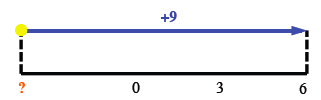Home > CC1MN > Chapter cc13 > Lesson cc13.2.3 > Problem3-119

3-119.

Solve the number puzzles below. 3-119 HW eTool (CPM)   Homework Help ✎

1. If I add $9$ to my number, I get $6$. What is my number?Based on the number line, what was the original number?

2. If I start at $-5$ on a number line and end up at $-8$, what direction did I move? How many units did I move?

Experiment with the number line on the eTool below and use your observations to answer the question.

3. If I moved up $8$ and then moved down $8$, what can you tell me about my ending position?

• Try making your own number line (this one should be vertical).
Draw arrows $8$ up and then $8$ down and see where you land.

It is the same as the starting position. Can you see why?

Use the eTool below to help you with this problem.
Click the link at right for the full version of the eTool: CC1 3-119 HW eTool